Courses

# Power Plant

## 25 Questions MCQ Test GATE Mechanical (ME) 2022 Mock Test Series | Power Plant

Description
This mock test of Power Plant for GATE helps you for every GATE entrance exam. This contains 25 Multiple Choice Questions for GATE Power Plant (mcq) to study with solutions a complete question bank. The solved questions answers in this Power Plant quiz give you a good mix of easy questions and tough questions. GATE students definitely take this Power Plant exercise for a better result in the exam. You can find other Power Plant extra questions, long questions & short questions for GATE on EduRev as well by searching above.
QUESTION: 1

### Common data for 1 & 2 Air enters the compressor of an ideal air standard Brayton cycle at 100 kPa, 200C and the pressure ratio across the compressor is 12:1.  Flow rate of air is 10 kg/s and maximum temperature in cycle is 11000C. Q. Compressor Power input is:-

Solution:

P2/P1 = 12.

then T2= (12)^{0..4/1.4} × 293 = 595.94K.

Then in ideal brayton cycle work done by compressor is =(h2- h1)= m×Cp× (T2-T1)= 10×1.005× (595.94-293) = 3044.54 KW = 3.04 MW

QUESTION: 2

### Common data for 1 & 2 Air enters the compressor of an ideal air standard Brayton cycle at 100 kPa, 200C and the pressure ratio across the compressor is 12:1. Flow rate of air is 10 kg/s and maximum temperature in cycle is 11000C. Q. Turbine Power output is:-

Solution: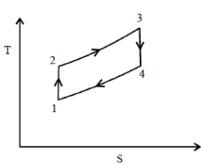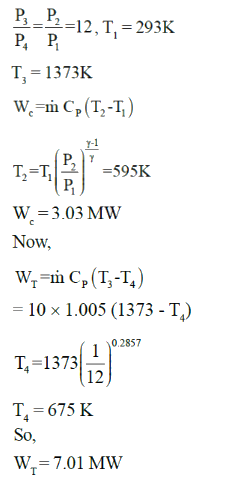QUESTION: 3

### Common data for 3 & 4 Brayton cycle has compression ratio of 16:1. Cycle produces 14 MW. Inlet state is 100 kPa, 170C. Heat added in combustion is 960 kJ/kg. Q. Highest temperature will be:-

Solution:
QUESTION: 4

Common data for 3 & 4

Brayton cycle has compression ratio of 16:1. Cycle produces 14 MW. Inlet state is 100 kPa, 170C. Heat added in combustion is 960 kJ/kg.

Q. Mass flow rate of air will be :-

Solution: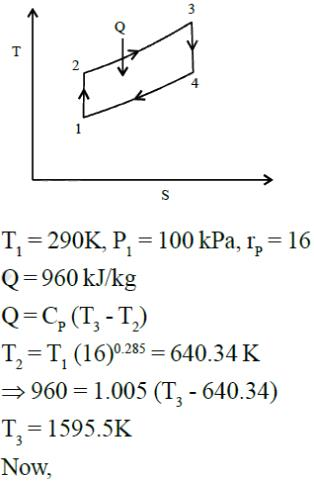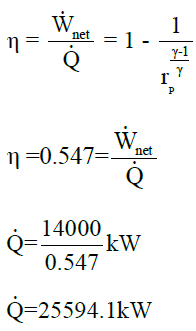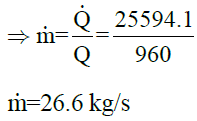QUESTION: 5

Temperature limits in an ideal air standard Brayton cycle are 300 K and 1500K. Pressure ratio is such that it maximises the net work developed by the cycle per unit mass of air flow. Thermal efficiency of cycle is:-

Solution: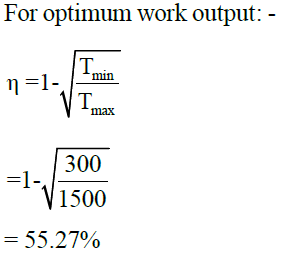QUESTION: 6

Compressor inlet temperature in ideal Brayton cycle is T1, turbine inlet temperature is T3. For maximum net work developed per unit mass of air flow, the temperature T2 at the compressor exit will be:-

Solution: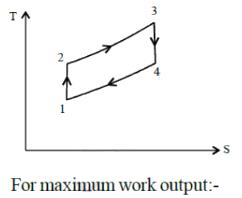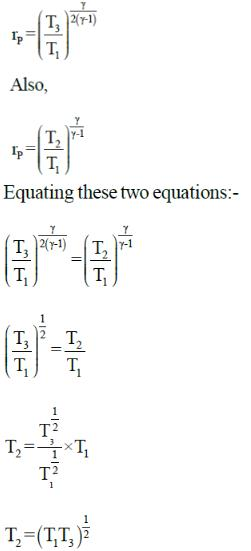QUESTION: 7

Brayton cycle operates between temperature limits of 270C and 7270C. Air is the working fluid. Maximum and minimum cycle pressure are 2000 kPa and 100 kPa respectively. Net work produced by this cycle is:-

Solution: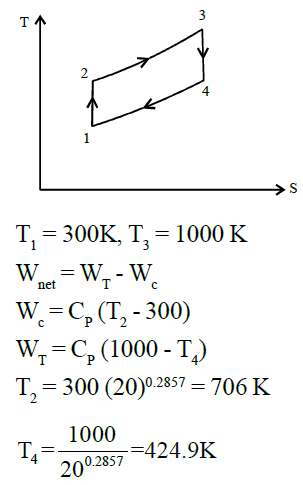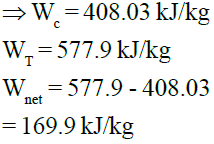QUESTION: 8

A simple Brayton Cycle has a pressure ratio of 10. The air at the beginning of compressor is at 70 kPa and 00C. Heat is added to the cycle at a rate of 500 kW and air passes at the rate of 1kg/s.
Power produced by this cycle is:-

Solution: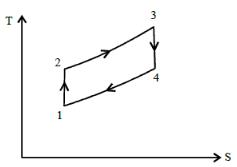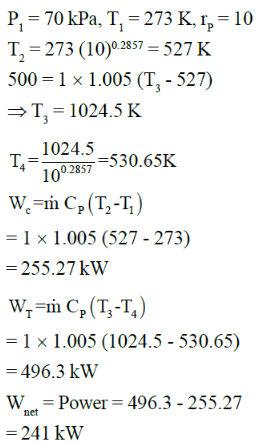QUESTION: 9

Brayton cycle operates between temperature limits of 300K and 1600 K. Power output is 100 MW.
The compressor pressure ratio is 14 to 1.

Thermal efficiency of cycle is:-

Solution: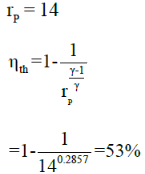QUESTION: 10

Carnot cycle operates between pressure and temperature limits of 20 kPa to 2000 kPa and 300 K to 900K respectively. If cycle executed in a closed system with 0.003 kg of air. Net work output per cycle will be:-

Solution: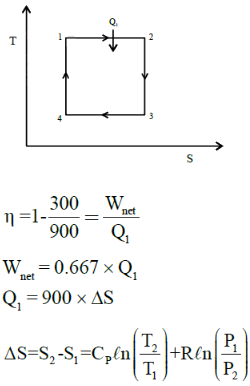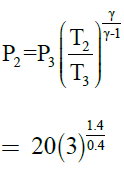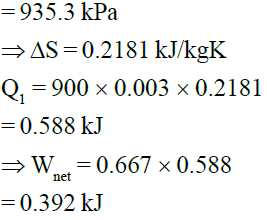QUESTION: 11

Carnot cycle uses heluim (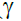= 1.667) as working fluid and rejects heat to lake at 150C. If thermal efficiency of cycle is to be 50%, the compression ratio will be:

Solution: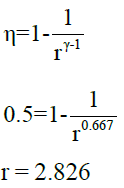QUESTION: 12

Ideal gas carnot cycle receives heat at 10270C. The cycle is repeated 1500 times per minute and has compression ratio of 12. Device produces power of 500 kW, the amount of heat supplied per cycle must be:-

Solution: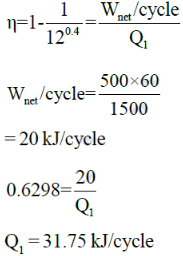QUESTION: 13

Common data for 13 & 14
Simple ideal Rankine Cycle has water as working Fluid. Cycle operates between 15 MPa in boiler and 100 kPa in condenser. Saturated steam enters turbine and steam quality at outlet of turbine is 70%

Q. Thermal efficiency of cycle is:-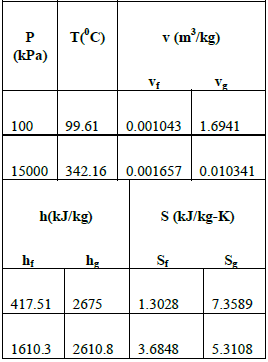Solution:
QUESTION: 14

Common data for 13 & 14
Simple ideal Rankine Cycle has water as working Fluid. Cycle operates between 15 MPa in boiler and 100 kPa in condenser. Saturated steam enters turbine and steam quality at outlet of turbine is 70%Q. Isentropic efficiency of turbine is:-

Solution: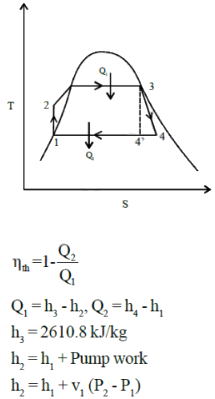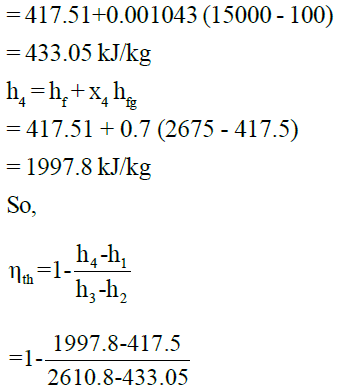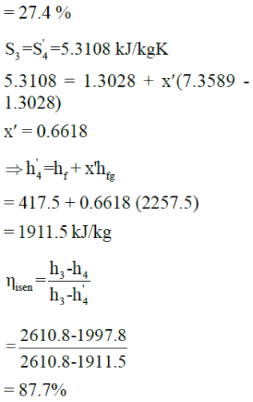QUESTION: 15

High pressure in Rankine cycle is determined in :-

Solution:

High pressure is determined by pump whereas low pressure is determined as saturation pressure for temperature. We can cool to in condenser

QUESTION: 16

Superheated vapour enters the turbine at 80bar, 4800C in an ideal Rankine cycle and condenser pressure in cycle is 0.08 bar. Net power output of cycle is 100 MW.

Consider the following table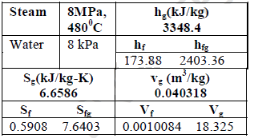Rate of heat transfer to working fluid passing through steam generator (in MW) is:-

Solution: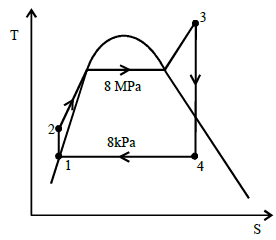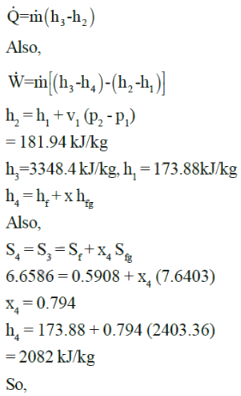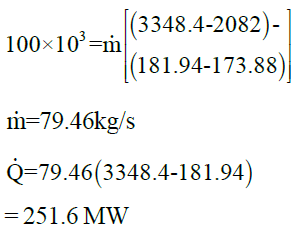QUESTION: 17

Common data for 17 & 18
In ideal Rankine cycle, steam enters turbine at 30 MPa, 5500C and exit at a pressure of 5kPa. It enters
condenser and exits as saturated water. Pump feeds back the water to boiler.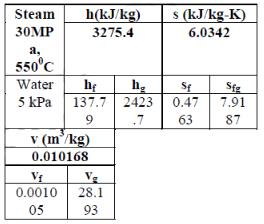Q. Steam quality at turbine exit is:-

Solution:
QUESTION: 18

Common data for 17 & 18
In ideal Rankine cycle, steam enters turbine at 30 MPa, 5500C and exit at a pressure of 5kPa. It enters
condenser and exits as saturated water. Pump feeds back the water to boiler.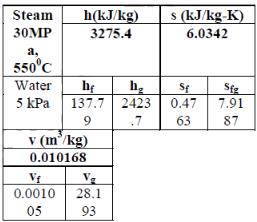Q. Thermal efficiency of cycle is:-

Solution: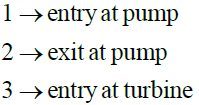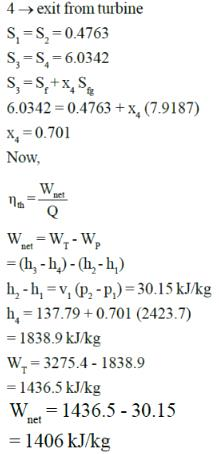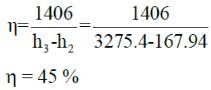QUESTION: 19

Simple ideal Rankine cycle use water as working fluid and operates between pressure limits of 3MPa in
boiler (h = 3115.5kJ/kg) and 30 kPa in condenser (hf = 289.27 kJ/kg, hfg = 2335.3 kJ/kg, v = 0.001022 m3/kg). If quality at exit of turbine cannot be less than 85%, maximum thermal efficiency of cycle is :-

Solution: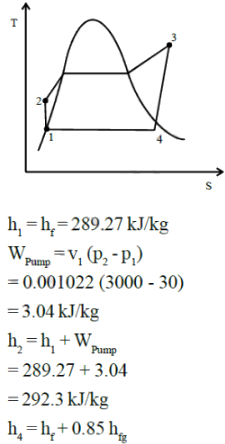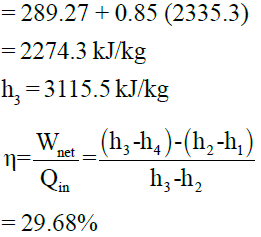QUESTION: 20

Consider a simple ideal Rankine cycle. If condenser pressure is lowered while keeping turbine inlet state the same.

Solution:

Area under the curve representing condenser decreases, so amount of heat rejected will decrease.

QUESTION: 21

Simple ideal Brayton cycle works between temperature limits of 300C and 8270C. Pressure ratio is 18 and
minimum cycle pressure is 100 kPa. Efficiency of this cycle is:-

(use standard value for air)

Solution: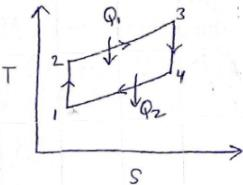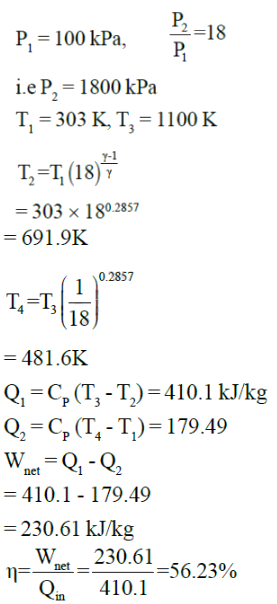QUESTION: 22

In a simple Brayton cycle, air has a pressure and temperature of 90 kPa and 100C before compression. It
has a pressure ratio of 10. Mass flow rate of air is 2 kg/s. Value of power produced by cycle is (heat is
added at rate of 600 kW):-

Solution: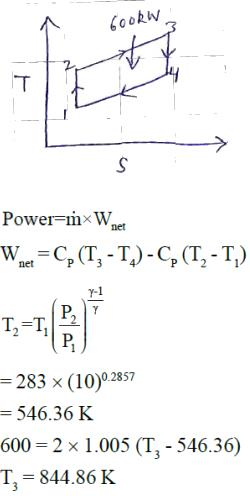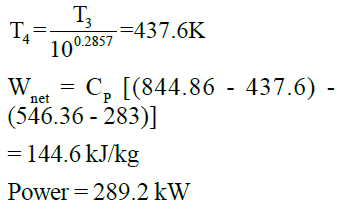QUESTION: 23

Thermal efficiency in previous question is given by :-

Solution: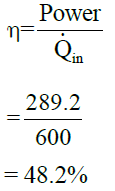QUESTION: 24

Maximum and minimum temperature in air standard gas turbine cycle are 1000 K and 200K. For maximum net work output, value of pressure ratio is:-

Solution: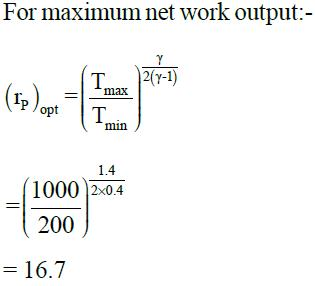QUESTION: 25

The isentropic enthalpy drop in moving blade is two-third of the isentropic enthalpy drop in fixed blades of a turbine. The degree of reaction will be

Solution:

Degree of reaction = isentropic enthalpy drop in moving blade/(isentropic enthalpy drop in fixed blade+isentropic enthalpy drop in moving blade).

= (2/3) / [1+(2/3)].

= (2/3) / (5/3),

= 2/5,

DOR = 0.4